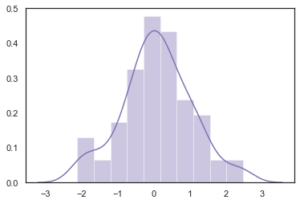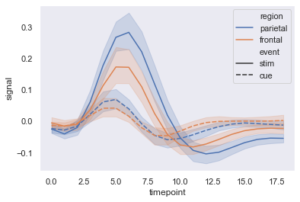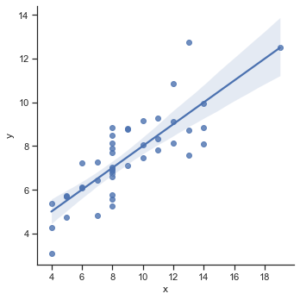Open in App
Not now

# Introduction to Seaborn – Python

• Difficulty Level : Basic
• Last Updated : 03 Jun, 2020

Seaborn is an amazing visualization library for statistical graphics plotting in Python. It provides beautiful default styles and color palettes to make statistical plots more attractive. It is built on the top of matplotlib library and also closely integrated to the data structures from pandas.
Seaborn aims to make visualization the central part of exploring and understanding data. It provides dataset-oriented APIs, so that we can switch between different visual representations for same variables for better understanding of dataset.

## Different categories of plot in Seaborn

Plots are basically used for visualizing the relationship between variables. Those variables can be either be completely numerical or a category like a group, class or division. Seaborn divides plot into the below categories –

• Relational plots: This plot is used to understand the relation between two variables.
• Categorical plots: This plot deals with categorical variables and how they can be visualized.
• Distribution plots: This plot is used for examining univariate and bivariate distributions
• Regression plots: The regression plots in seaborn are primarily intended to add a visual guide that helps to emphasize patterns in a dataset during exploratory data analyses.
• Matrix plots: A matrix plot is an array of scatterplots.
• Multi-plot grids: It is an useful approach is to draw multiple instances of the same plot on different subsets of the dataset.

### Installation

For python environment :

```pip install seaborn
```

For conda environment :

```conda install seaborn
```

### Dependencies

• Python 3.6+
• numpy (>= 1.13.3)
• scipy (>= 1.0.1)
• pandas (>= 0.22.0)
• matplotlib (>= 2.1.2)
• statsmodel (>= 0.8.0)

## Some basic plots using seaborn

Dist plot :  Seaborn dist plot  is used to plot a histogram, with some other variations like kdeplot and rugplot.

## Python3

 `# Importing libraries ` `import` `numpy as np ` `import` `seaborn as sns ` ` `  ` `  `# Selecting style as white, ` `# dark, whitegrid, darkgrid  ` `# or ticks ` `sns.``set``(style``=``"white"``) ` ` `  `# Generate a random univariate  ` `# dataset ` `rs ``=` `np.random.RandomState(``10``) ` `d ``=` `rs.normal(size``=``100``) ` ` `  `# Plot a simple histogram and kde  ` `# with binsize determined automatically ` `sns.distplot(d, kde``=``True``, color``=``"m"``)`

Output:Line plot : The line plot is one of the most basic plot in seaborn library.  This plot is mainly used to visualize the data in form of some time series, i.e. in continuous manner.

## Python3

 `import` `seaborn as sns ` ` `  ` `  `sns.``set``(style``=``"dark"``) ` `fmri ``=` `sns.load_dataset(``"fmri"``) ` ` `  `# Plot the responses for different\ ` `# events and regions ` `sns.lineplot(x``=``"timepoint"``, ` `             ``y``=``"signal"``, ` `             ``hue``=``"region"``, ` `             ``style``=``"event"``, ` `             ``data``=``fmri)`

Output :Lmplot :  The lmplot is another most basic plot. It shows a line representing a linear regression model along with data points on the 2D-space and x and y can be set as the horizontal and vertical labels respectively.

## Python3

 `import` `seaborn as sns ` ` `  `sns.``set``(style``=``"ticks"``) ` ` `  `# Loading the dataset ` `df ``=` `sns.load_dataset(``"anscombe"``) ` ` `  `# Show the results of a linear regression ` `sns.lmplot(x``=``"x"``, y``=``"y"``, data``=``df)`

Output :My Personal Notes arrow_drop_up
Related Articles